• ## 曲线平滑算法

万次阅读 2018-08-10 23:57:01
由于项目开发需要对等值线进行平滑处理，所以研究了线条的平滑算法，经研究查阅资料，可以使用三次B样条曲线方程对线条进行平滑处理，而平滑处理可分为近似拟合和插值拟合两种，两种拟合处理各有其优缺点，以下会做...
由于项目开发需要对等值线进行平滑处理，所以研究了线条的平滑算法，经研究查阅资料，可以使用三次B样条曲线方程对线条进行平滑处理，而平滑处理可分为近似拟合和插值拟合两种，两种拟合处理各有其优缺点，以下会做说明，可根据实际业务需要进行选取。本次项目开发最终选用了近似拟合算法处理。

一、三次B样条曲线方程

B样条曲线的总方程为：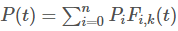其中是控制曲线的特征点，则是K阶B样条基函数。

三次B样条曲线方程中基函数为：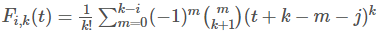其中表示阶乘，化成看的明白的式子就是：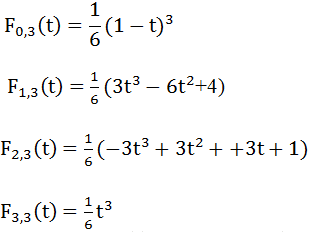将基函数带入总方程可换算出三次B样条曲线方程：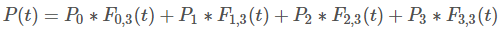基于上述的三次B样条曲线方程可对曲线进行平滑处理。

二、三次B样条曲线近似拟合

近似拟合，平滑后的线条不一定过原始特征点，平滑处理的核心算法如下，算法中对闭合曲线和非闭合曲线分别做了处理：

// 闭合曲线
if (line == line[len - 2] && line == line[len - 1]) {
for (var n = 0; n < pntLen; n++) {
if (n <= pntLen - 4) {
for (var t = 0.0; t <= 1.0; t += 0.1) {
var a1 =  Math.pow((1 - t), 3) / 6;
var a2 =  (3 * Math.pow(t, 3) - 6 * Math.pow(t, 2) + 4) / 6;
var a3 = (-3 * Math.pow(t, 3) + 3 * Math.pow(t, 2) + 3 * t + 1) / 6;
var a4 =  Math.pow(t, 3) / 6;

var x = a1*points[n].x + a2*points[n + 1].x + a3*points[n + 2].x + a4*points[n + 3].x;
var y = a1*points[n].y + a2*points[n + 1].y + a3*points[n + 2].y + a4*points[n + 3].y;
newPnts.push({x: x, y: y});
}
} else if (n == pntLen - 3) {
for (var t = 0.0; t <= 1.0; t += 0.1) {
var a1 =  Math.pow((1 - t), 3) / 6;
var a2 =  (3 * Math.pow(t, 3) - 6 * Math.pow(t, 2) + 4) / 6;
var a3 = (-3 * Math.pow(t, 3) + 3 * Math.pow(t, 2) + 3 * t + 1) / 6;
var a4 =  Math.pow(t, 3) / 6;

var x = a1*points[n].x + a2*points[n + 1].x + a3*points[n + 2].x + a4*points.x;
var y = a1*points[n].y + a2*points[n + 1].y + a3*points[n + 2].y + a4*points.y;
newPnts.push({x: x, y: y});
}
} else if (n == pntLen - 2) {
for (var t = 0.0; t <= 1.0; t += 0.1) {
var a1 =  Math.pow((1 - t), 3) / 6;
var a2 =  (3 * Math.pow(t, 3) - 6 * Math.pow(t, 2) + 4) / 6;
var a3 = (-3 * Math.pow(t, 3) + 3 * Math.pow(t, 2) + 3 * t + 1) / 6;
var a4 =  Math.pow(t, 3) / 6;

var x = a1*points[n].x + a2*points[n + 1].x + a3*points.x + a4*points.x;
var y = a1*points[n].y + a2*points[n + 1].y + a3*points.y + a4*points.y;
newPnts.push({x: x, y: y});
}
} else if (n == pntLen - 1) {
for (var t = 0.0; t <= 1.0; t += 0.1) {
var a1 =  Math.pow((1 - t), 3) / 6;
var a2 =  (3 * Math.pow(t, 3) - 6 * Math.pow(t, 2) + 4) / 6;
var a3 = (-3 * Math.pow(t, 3) + 3 * Math.pow(t, 2) + 3 * t + 1) / 6;
var a4 =  Math.pow(t, 3) / 6;

var x = a1*points[n].x + a2*points.x + a3*points.x + a4*points.x;
var y = a1*points[n].y + a2*points.y + a3*points.y + a4*points.y;
newPnts.push({x: x, y: y});
}
}
}
// 不闭合
} else {
newPnts.push({x: points.x, y: points.y});
for (var n = 0; n < pntLen; n++) {
if (n <= pntLen - 4) {
for (var t = 0.0; t <= 1.0; t += 0.1) {
var a1 =  Math.pow((1 - t), 3) / 6;
var a2 =  (3 * Math.pow(t, 3) - 6 * Math.pow(t, 2) + 4) / 6;
var a3 = (-3 * Math.pow(t, 3) + 3 * Math.pow(t, 2) + 3 * t + 1) / 6;
var a4 =  Math.pow(t, 3) / 6;

var x = a1*points[n].x + a2*points[n + 1].x + a3*points[n + 2].x + a4*points[n + 3].x;
var y = a1*points[n].y + a2*points[n + 1].y + a3*points[n + 2].y + a4*points[n + 3].y;
newPnts.push({x: x, y: y});
}
}
}
newPnts.push({x: points[pntLen - 1].x, y: points[pntLen - 1].y});
}

三次B样条曲线近似拟合处理的效果图如下，其中红色为原始未平滑处理的曲线，而蓝色为平滑后的曲线。可以看出近似拟合后的曲线平滑的效果较为满意，但是缺点是曲线不过原始特征点 。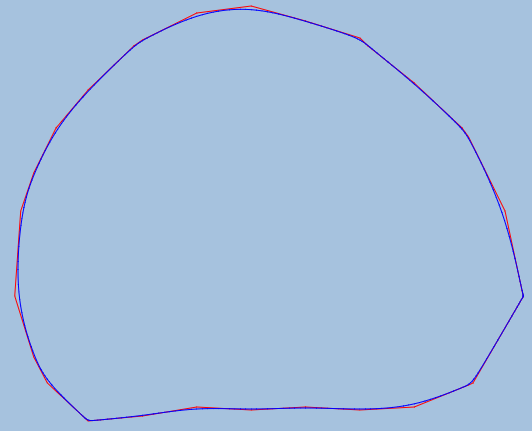三、三次B样条曲线插值拟合

插值拟合，平滑后的曲线过原始特征点，平滑的核心算法如下：

function getCtrlPoint(pointsArr, index, scaleA, scaleB) {
if (!scaleA || !scaleB) {
scaleA = 0.15;
scaleB = 0.15;
}

// 处理两种极端情形
if (index < 1) {
var pAx = pointsArr.x + (pointsArr.x - pointsArr.x) * scaleA;
var pAy = pointsArr.y + (pointsArr.y - pointsArr.y) * scaleA;
} else {
var pAx = pointsArr[index].x + (pointsArr[index + 1].x - pointsArr[index - 1].x) * scaleA;
var pAy = pointsArr[index].y + (pointsArr[index + 1].y - pointsArr[index - 1].y) * scaleA;
}

if (index > pointsArr.length - 3) {
var last = pointsArr.length - 1
var pBx = pointsArr[last].x - (pointsArr[last].x - pointsArr[last - 1].x) * scaleB;
var pBy = pointsArr[last].y - (pointsArr[last].y - pointsArr[last - 1].y) * scaleB;
} else {
var pBx = pointsArr[index + 1].x - (pointsArr[index + 2].x - pointsArr[index].x) * scaleB;
var pBy = pointsArr[index + 1].y - (pointsArr[index + 2].y - pointsArr[index].y) * scaleB;
}

return {
pA: {x: pAx, y: pAy},
pB: {x: pBx, y: pBy}
}
}

三次B样条曲线插值拟合处理的效果图如下，其中红色为原始未平滑处理的曲线，而蓝色为平滑后的曲线。可以看出插值拟合后的曲线平滑的效果不尽如人意，有出现线条打结的情况，但是优点是曲线过原始特征点 。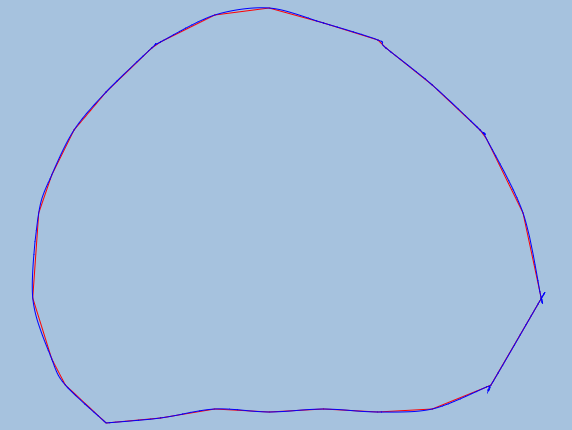参考资料：https://blog.csdn.net/liumangmao1314/article/details/54588155 
展开全文三次贝塞尔曲线 JavaScript
• 详细源码讲解如何计算贝塞尔曲线，可以实现曲线平滑算法
• 基于热敏打印的心电曲线平滑算法的研究及实现，对于使用热敏打印机打印曲线有很好的参考和指导作用。
• bezier曲线平滑算法研究一、Bezier曲线定义：给定n+1个控制顶点Pi(i=0~n) ，则Bezier曲线定义为：P(t)=∑Bi,n(t)Pi u∈[0,1]其中：Bi,n(t)称为基函数。Bi,n(t)=Ci nti (1-t)n-iCi n=n!/(i!*(n-i)!)二、Bezier曲线...
 bezier曲线平滑算法研究
一、Bezier曲线定义：给定n+1个控制顶点Pi(i=0~n) ，则Bezier曲线定义为：P(t)=∑Bi,n(t)Pi u∈[0,1]其中：Bi,n(t)称为基函数。Bi,n(t)=Ci nti (1-t)n-iCi n=n!/(i!*(n-i)!)
二、Bezier曲线性质1、端点性质：a）P(0)=P0, P(1)=Pn, 即：曲线过二端点。b）P’(0)=n(P1-P0), P’(1)=n(Pn-Pn-1)即：在二端点与控制多边形相切。2、凸包性：Bezier曲线完成落在控制多边形的凸包内。3、对称性：由Pi与Pn-i组成的曲线，位置一致，方向相反。4、包络性：Pn (t)=(1-t)Pn-1 (t)+tPn-1 (t)

编程语言 C++
#include<stdio.h>
void Bezier(int x1,int y1,int z1,int x2,int y2,int z2,int x3,int y3,int z3,int x4,int y4,int z4,float u){ double a,b,c;a=x1;b=y1;c=z1;a=x2;b=y2;c=z2;a=x3;b=y3;c=z3;a=x4;b=y4;c=z4;for(int i=1;i<=3;i++)for(int j=0;j<4-i;j++){a[i][j]=(1-u)*a[i-1][j]+u*a[i-1][j+1];b[i][j]=(1-u)*b[i-1][j]+u*b[i-1][j+1];c[i][j]=(1-u)*c[i-1][j]+u*c[i-1][j+1];}printf("%10lf%10lf%10lf/n",a,b,c);}
void main(){int x1,y1,z1,x2,y2,z2,x3,y3,z3,x4,y4,z4;float u;printf("输入Bezier曲线第控制顶点/n");scanf("%d%d%d%d%d%d%d%d%d%d%d%d",&x1,&y1,&z1,&x2,&y2,&z2,&x3,&y3,&z3,&x4,&y4,&z4);for(;;){fflush(stdin);printf("输入u=");scanf("%f",&u);Bezier(x1,y1,z1,x2,y2,z2,x3,y3,z3,x4,y4,z4,u);}}

展开全文float c
• Interpolation with Bezier Curves 贝塞尔插值A very simple method of smoothing polygons 一种非常简单的多边形平滑方法翻译：唐风之前 comp.graphic.algorithms 上有一个讨论，是关于怎么样使用曲线对多边形进行...
Interpolation with Bezier Curves  贝塞尔插值A very simple method of smoothing polygons 一种非常简单的多边形平滑方法翻译：唐风之前 comp.graphic.algorithms 上有一个讨论，是关于怎么样使用曲线对多边形进行插值处理，使得最终产生的曲线是光滑的而且能通过所有的顶点。Gernot Hoffmann 建议说使用著名的 B-Spline 来进行插值。这里有他当时的文章。B-Spline 在这里效果很好，它看起来就像是一个固定在多边形顶点上的橡皮尺(elastic ruler)。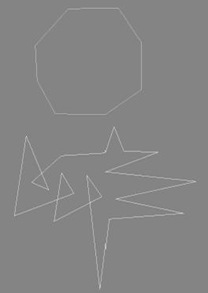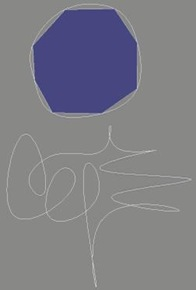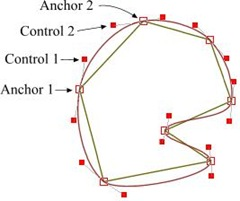但我有个大胆的推测，我觉得肯定还存在更简单的方法。比如，使用三次贝塞曲线(cubic Bezier)进行近似。贝塞尔曲线有两个固定点(起点和终点)，另加两个决定曲线形状的控制点(CP)。关于贝塞尔曲线的更多知识可以在搜索引擎中找到，比如，你可以参考Paul Bourke 的站点。 现在给贝塞尔曲线的锚点(固定点)，也就是多边形的某一对顶点，那么问题是，我们怎么计算控制点的位置？我运行 Xara X 然后画出了右边这个图形，这很简单，所以我决定尝试下计算出它们的坐标。很显然，多边形两条相邻边的两个控制点与这两个控制点之间的顶点应该在一条直线 上，只有这样，两条相邻的插值曲线才能平滑地连接在一起。所以，这两个控制点应该是相对于顶点是对称的，不过，也不全是……，因为真正的对称就要求它们与 中心点的距离应该是相等的，但对于我们的情况中并不完全是这样的。一开始，我试着先算出多边形两条边的角平分线，然后把控制点放在这条角平分线的垂直线 上。但从图上可以看到，控制点的连线并不会总是垂直于角平分线的。最终，我找到一个非常简单的办法，不需要任何复杂的数学计算。首先，我们计算出多边形所有边线的中点，Ai。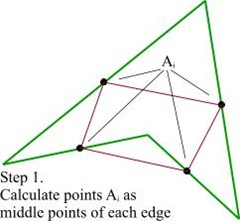然后连接起相邻边中点，得到很多线段，记为 Ci 。并用图记的方法计算出 Bi 点。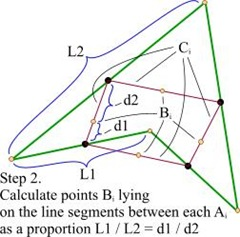最后一步，只需要简单地将 Ci 进行平移，平移的路径就是每条线段上 Bi 到对应顶点的路径。就这样，我们计算出了贝塞尔曲线的控制点，平滑的结果看起来也很棒。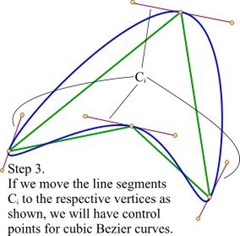这里还可以做一点小小的改进，因为我们已经得到了一条决定控制点的直线，所以，我们可以根据需要，使控制点在这条直线上移动，这样可以改变插值曲线 的状态。我使用了一个与控制点和顶点初始距离相关的系数 K ，用来沿直线移动控制点。控制点离顶点越远，图形看起来就越锐利。下面是用原始形状和系统K=1.0的贝塞尔插值两种方法来描画的 SVG 的狮子。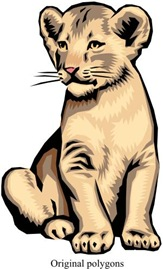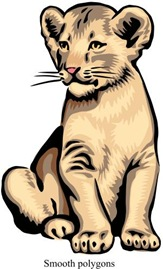下面是放大图这个方法对于自相关的多边形也适用，下面的例子可以看到，结果非常有意思：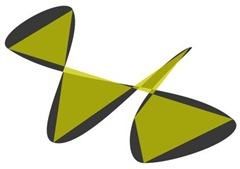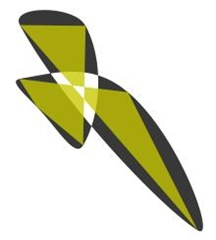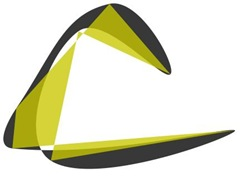这个方法只是探索和经验式的，如果从严格的数学模型的角度看它可能是错误的。但在实际使用中的效果已经足够好了，而 且这个方法只需要最小的计算量。下面的代码就是用来画出上面狮子图像的。这些代码并没有进行优化，只是用来演示的。里面有些变量计算了两次，在实际程序 中，如果连续的步骤中都用到同一个变量值，我们可以先缓存变量值进行复用(以避免重复的计算)。This method is pure heuristic and empiric. It probably givesa wrong result from the point of view of strict mathematicalmodeling. But in practice the result is good enough and itrequires absolute minimum of calculations. Below is the source codethat has been used to generate the lions shown above. It'snot optimal and just an illustration. It calculates some variablestwice, while in real programs we can store and reuse them in the// Assume we need to calculate the control// points between (x1,y1) and (x2,y2).// Then x0,y0 - the previous vertex,//      x3,y3 - the next one.doublexc1 = (x0 + x1) / 2.0;doubleyc1 = (y0 + y1) / 2.0;doublexc2 = (x1 + x2) / 2.0;doubleyc2 = (y1 + y2) / 2.0;doublexc3 = (x2 + x3) / 2.0;doubleyc3 = (y2 + y3) / 2.0;doublelen1 = sqrt((x1-x0) * (x1-x0) + (y1-y0) * (y1-y0));doublelen2 = sqrt((x2-x1) * (x2-x1) + (y2-y1) * (y2-y1));doublelen3 = sqrt((x3-x2) * (x3-x2) + (y3-y2) * (y3-y2));doublek1 = len1 / (len1 + len2);doublek2 = len2 / (len2 + len3);doublexm1 = xc1 + (xc2 - xc1) * k1;doubleym1 = yc1 + (yc2 - yc1) * k1;doublexm2 = xc2 + (xc3 - xc2) * k2;doubleym2 = yc2 + (yc3 - yc2) * k2;// Resulting control points. Here smooth_value is mentioned// above coefficient K whose value should be in range [0...1].ctrl1_x = xm1 + (xc2 - xm1) * smooth_value + x1 - xm1;ctrl1_y = ym1 + (yc2 - ym1) * smooth_value + y1 - ym1;ctrl2_x = xm2 + (xc2 - xm2) * smooth_value + x2 - xm2;ctrl2_y = ym2 + (yc2 - ym2) * smooth_value + y2 - ym2;consecutive steps.使用三次贝塞尔近似的代码：// Number of intermediate points between two source ones,// Actually, this value should be calculated in some way,// Obviously, depending on the real length of the curve.// But I don't know any elegant and fast solution for this// problem.#define NUM_STEPS 20voidcurve4(Polygon* p,doublex1,doubley1,//Anchor1doublex2,doubley2,//Control1doublex3,doubley3,//Control2doublex4,doubley4)//Anchor2{doubledx1 = x2 - x1;doubledy1 = y2 - y1;doubledx2 = x3 - x2;doubledy2 = y3 - y2;doubledx3 = x4 - x3;doubledy3 = y4 - y3;doublesubdiv_step  = 1.0 / (NUM_STEPS + 1);doublesubdiv_step2 = subdiv_step*subdiv_step;doublesubdiv_step3 = subdiv_step*subdiv_step*subdiv_step;doublepre1 = 3.0 * subdiv_step;doublepre2 = 3.0 * subdiv_step2;doublepre4 = 6.0 * subdiv_step2;doublepre5 = 6.0 * subdiv_step3;doubletmp1x = x1 - x2 * 2.0 + x3;doubletmp1y = y1 - y2 * 2.0 + y3;doubletmp2x = (x2 - x3)*3.0 - x1 + x4;doubletmp2y = (y2 - y3)*3.0 - y1 + y4;doublefx = x1;doublefy = y1;doubledfx = (x2 - x1)*pre1 + tmp1x*pre2 + tmp2x*subdiv_step3;doubledfy = (y2 - y1)*pre1 + tmp1y*pre2 + tmp2y*subdiv_step3;doubleddfx = tmp1x*pre4 + tmp2x*pre5;doubleddfy = tmp1y*pre4 + tmp2y*pre5;doubledddfx = tmp2x*pre5;doubledddfy = tmp2y*pre5;intstep = NUM_STEPS;// Suppose, we have some abstract object Polygon which// has method AddVertex(x, y), similar to LineTo in// many graphical APIs.// Note, that the loop has only operation add!while(step--){fx   += dfx;fy   += dfy;dfx  += ddfx;dfy  += ddfy;ddfx += dddfx;ddfy += dddfy;p->AddVertex(fx, fy);}p->AddVertex(x4, y4); // Last step must go exactly to x4, y4}你可以下载一个能运行的画狮子的例子，对它进行旋转和缩放，也可以生成一些随机的多边形。点左键并拖动它可以围绕中 心点旋转和缩放图像。点右键并从左向右拖动，可以改变系统数K。 K＝1时大约是距窗口左边100像素处。每次双击会产生一个随机的多边形，对于这些多边形，也可以进行旋转、缩放以及改变K值的操作。
展开全文• 前言系统的可靠性，是用户使用的信心所在。在资源有限的情况下，面对不断增长的流量，...简单窗口流量曲线优点：实现简单缺点：精确度不够，临界情况下会qps会超出实际的值。滑动窗口简单来看，简单窗口精确度不够...
前言系统的可靠性，是用户使用的信心所在。在资源有限的情况下，面对不断增长的流量，如何保证系统依然处于一个正常负载的状态呢？限流。限流问题可以追溯到网络通信中的流量整形(Traffic Shaping)和流量控制(Traffic Policing）。下面一起小窥一下有哪些精彩的算法。简单窗口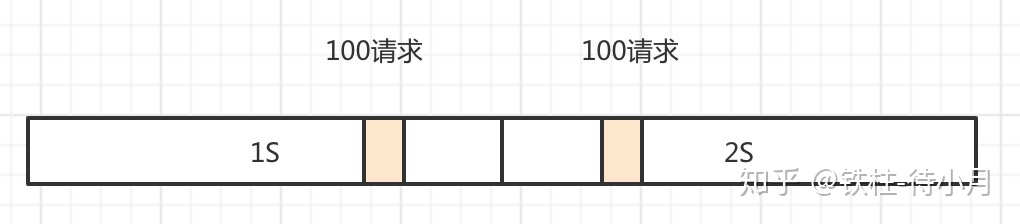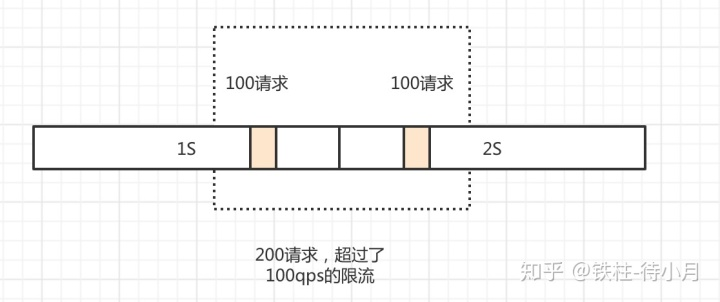流量曲线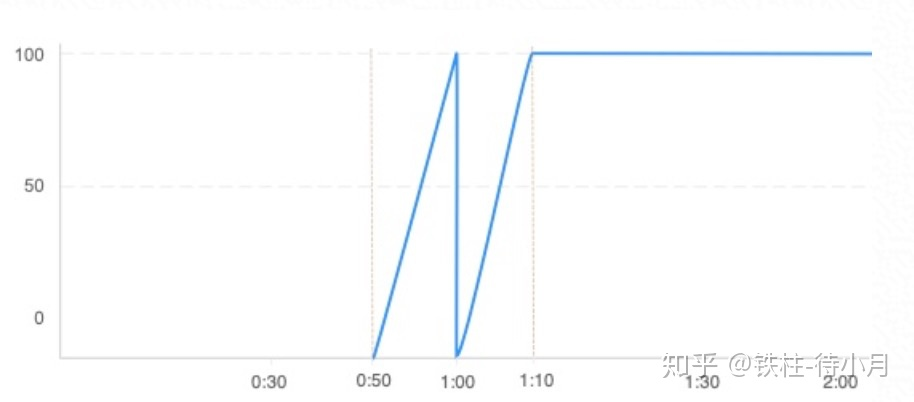优点：实现简单缺点：精确度不够，临界情况下会qps会超出实际的值。滑动窗口简单来看，简单窗口精确度不够的原因在于，窗口移动的步伐太大，没有关注到之前窗口的流量。导致漏掉了一些统计窗口，所以我们可以慢慢的移动，让过去的流量被关注到，避免漏掉统计窗口。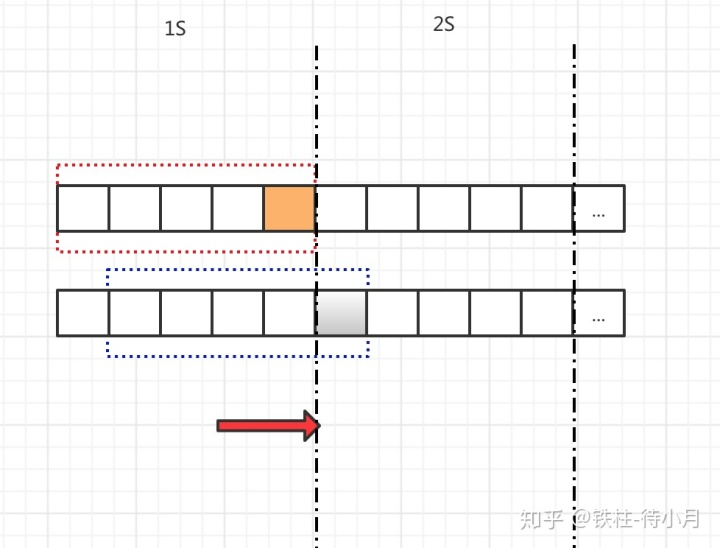但是这样就解决问题了吗？它只是概率性的缓解了问题。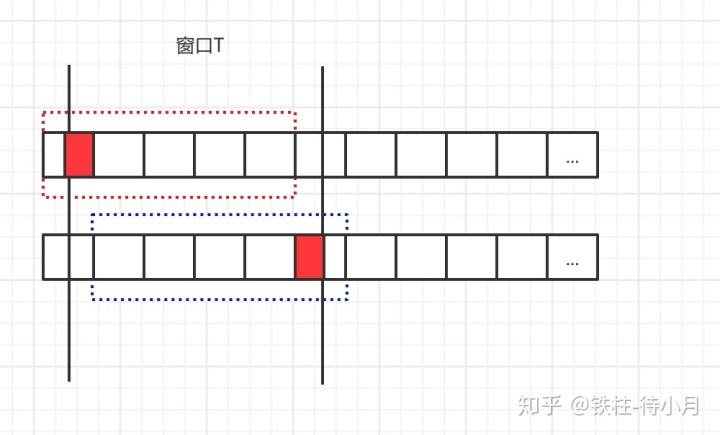相邻的窗口之间确实没有了突发流量，但是只要是有滑动距离且滑动距离内可以进入多个流量，就会像上图所示，在统计窗口T内超过本来的限流设置。流量曲线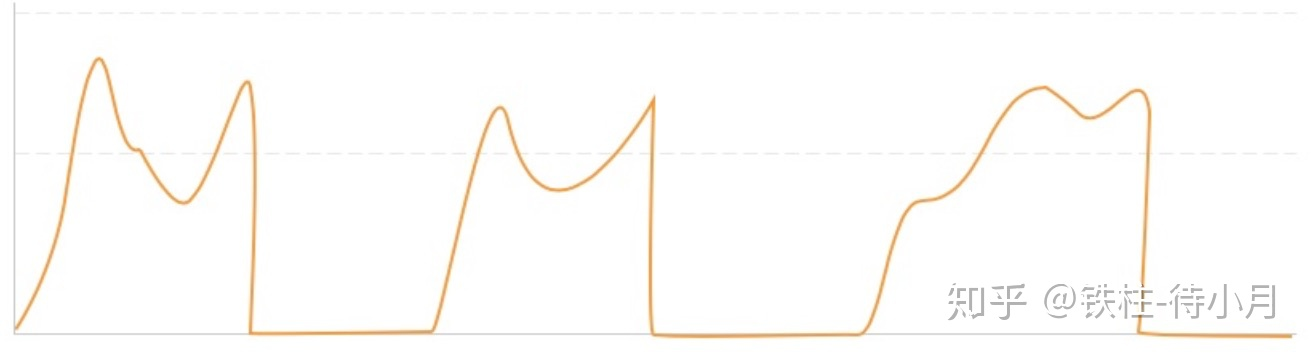优点：一定程度上解决了精确度的问题，缺点：精确度不稳定，极端情况下还是会超过总的qps（当然我们可以通过减小时间窗口来减少这种概率，但是不能完全消除掉，且增多时间窗口会导致计算复杂度升高，从而降低限流组件本身的性能）。滑动日志那么有办法保证绝对的精度吗？有！我们可以分析一下，之所以有精确度问题，是因为无论我们如何划分时间窗口，只要是一个静态划分且这个窗口可再分割（不涉及哲学问题和普朗克时间balabala），那么就存在我们关注不到的统计窗口。换句话说统计窗口几乎是无限的，难道这就结束了吗？我们换个思路再来看一下，其实我们的问题在于丢掉了统计窗口，我们到底丢掉了哪些统计窗口。然后你会发现我们丢掉的窗口都是因为人为划分了时间区域模糊掉了区域中请求的真正到达时间。也就是说我们其实不必追究统计窗口无限的这个点，只需要把这些被摸糊掉的请求到达时间记录下来并且每次进行统计窗口的判断就可以了。那么算法就演变成了当每次流控判断时，取出以当前请求时间为end的时间窗口内的请求数，看是否大于流控阈值。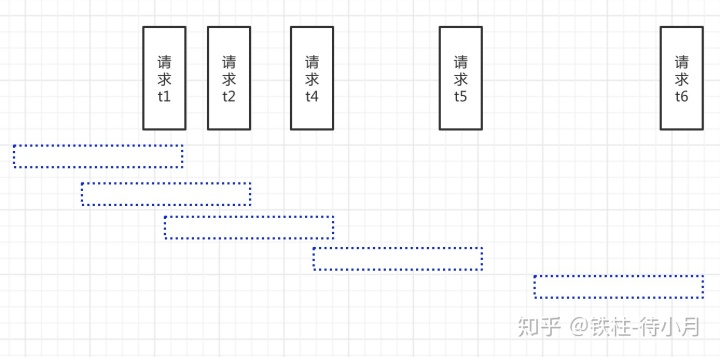这样每一个统计窗口都被我们关注到了，精确度问题解决。但是我们付出了计算复杂度的代价。优点：精确度完全精确。缺点：首先，我们要保存一个长度最大为N的请求日志队列，这意味着空间复杂度达到O(N)，如果要针对不同的流控key，那么空间上会占用更多。其次，我们需要在队列中确定时间窗口，即寻找不早于当前时间戳t-T的请求记录。以二分查找为例，时间复杂度是O(logN)。流量曲线整体的流量形状与滑动窗口的类似，只不过不会存在超过限流的情况，精确度提升。平滑度问题虽然我们付出了计算复杂度的代价，好在精确度问题有解了。这就结束了吗？还没有！如果你仔细观察这些算法的流量曲线的话，你会发现他们都有一个特点，就是尖刺凸起！尖刺凸起代表什么问题呢？比如设置的限流是100qps，但是这100个请求在窗口的前10ms就进来了，如果把这10ms内的请求折算为QPS的话，是100*(1000/10ms) = 10000qps，这个从请求数来看没什么变化，但是从负载来看确实远超设置的限流qps的。如果从监控的秒s级别来看流量曲线，是很平滑的。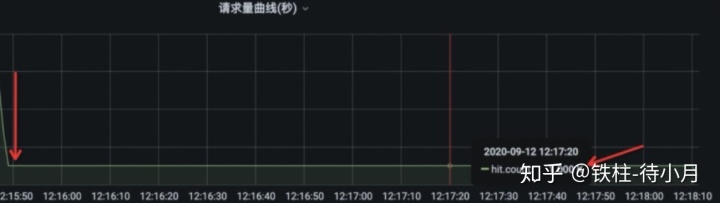但是如果你放大到毫秒ms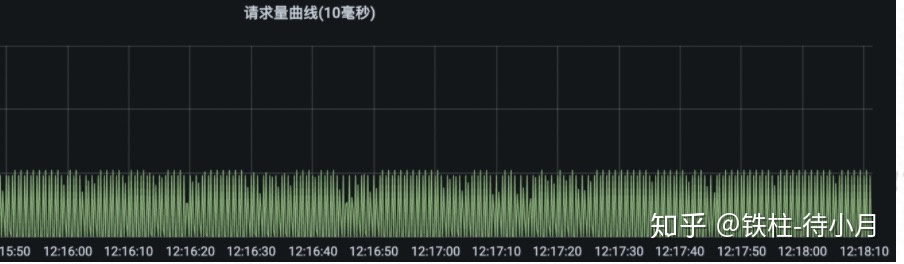再放大一点，你就发现跟上面的流量曲线很类似了。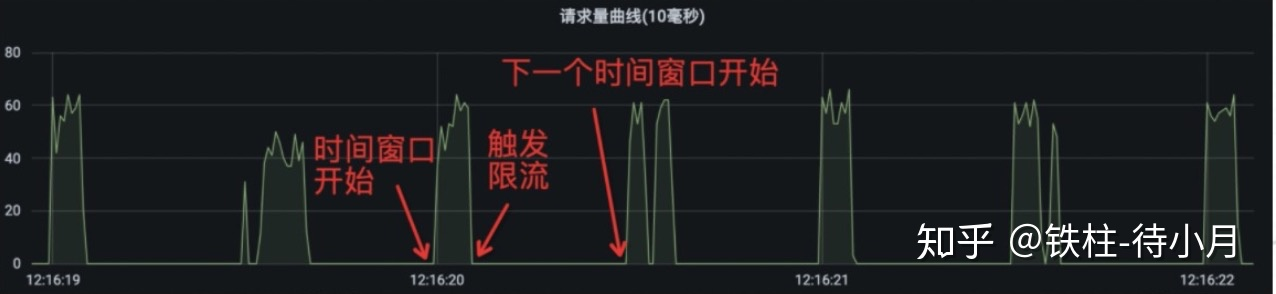问题在哪？？外部请求流量不是均匀的，因为我们只是按照窗口约束了窗口内的流量大小，但是对于窗口内是否均匀并没有定义。当然我们可以减小这个窗口，变成qpms,qpus,qpns，从而达到更细粒度的平滑，但是同时也伴随着更更细粒度的尖刺，因为即便是qpns级别，外部请求依然是可以在1ns的前十分之一处全部打进来，看起来我们可以用计算复杂度去换取平滑度，但是仿佛不能做到绝对平滑。漏桶&令牌桶那平滑度还有更好的办法吗？我们再来分析一下为什么不平滑， 其中有一个原因在于窗口的机制只是对窗口之间的平滑度做了限制，而没有限制窗口内，即便窗口分的再细，依然存在窗口内的小凸起。所以我们只要把窗口内也一同限制了就好了嘛！漏桶和令牌桶算法便是这样来平滑流量的，他们的基本思路就是控制平均访问速率v ≤ N / T。（在这里我就不把图贴上来了，网上有很多。）令牌桶：有一个人在匀速的按照速度v往桶里面放令牌直到桶满，然后请求者去取令牌，取到就算通过，否则限流。漏桶：有一个人在匀速的按照速度v往桶里面往外拿令牌，然后请求者去放令牌，桶里还能放得下就算通过，否则限流。个人认为在桶的容量是1时这两个算法本质是一样的。只不过在桶的容量不是1的时候，在流量曲线上会有一些不同。PS:当桶的容量大于1时，会和滑动窗口算法一样，有超过限流值的风险。令牌桶的容量大于1时的流量曲线：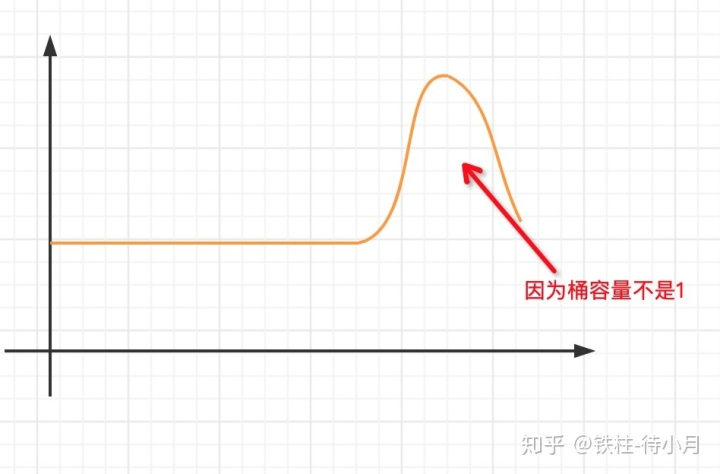漏桶的容量大于1时的流量曲线：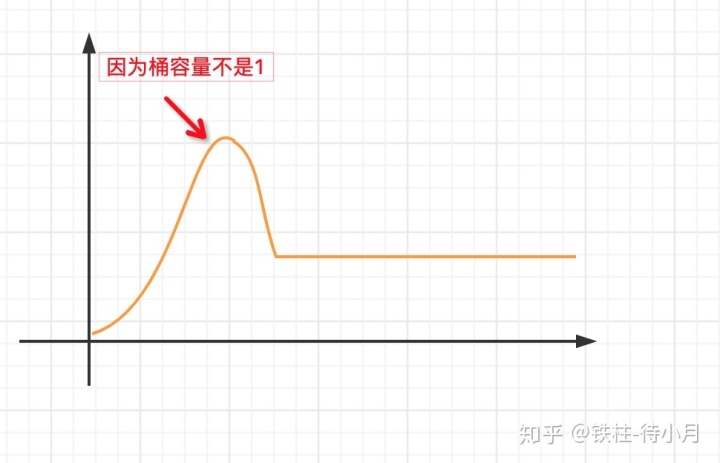优点：可控的平滑度，且当桶容量等于1时，精确度和平滑度都得到了完美的解决。（一点小问题-速率依赖于时间精度，时间精度会依赖于时钟物理上限，时间精度也是一种计算复杂度）缺点：当桶容量大于1时会有超过限流值的风险。虽然精确度和平滑度都解决了，但是遇到突发流量时会设计到队列排队问题甚至会大量拒绝请求，且用户的RT时间会变长，体验不好，很多业务对RT是相当敏感的。Sentinel的实现那Sentinel是如何实现流控的呢？Cumulative moving average在本文看来这是一种在子窗口内允许小尖刺，避免了大窗口内的大尖刺，从而满足平滑度的需求。是细化了窗口粒度的滑动窗口算法。一些优化细节：数据统计为了统计资源的访问次数，使用了加锁的HashMap 而不是 ConcurrentHashMap，因为程序只会在最开始的时候用一次锁，所以程序稳定以后HashMap性能会更好。时间获取：daemon线程定时更新当前时间窗口滑动：环形数组指标计数：LongAdder：不是绝对准确的，但sentinel的场景计数操作并不是核心，性能更优。指标计数时使用了返回累加的和可能不是绝对准确的，因为调用这个方法时还有其他线程可能正在进行计数累加，，这种情况使用LongAdder则是更优的一种考虑总结如果把上面这些算法抽象一下、你会发现限流问题变成了这样一句话：任意给定时间窗口T内的访问量不大于N，如何保证算法的精确度、平滑度、平均RT、计算复杂度。总体看来上面这些算法都是有缺憾的，因为这几个维度中精确度和计算复杂度是有技术矛盾的，而平滑度又和平均RT有技术矛盾。所以看起来在这四个维度都达到完美不是那么容易的，针对限流问题在这几个维度不同的解答和权衡，就得到了不同的限流算法。会有算法在这几个维度更接近于完美吗？不知道，我相信或许这就是技术的魅力所在的，追求是永无止境的。期待更多精巧的算法。附图有些是自己画的，有些是借用别人的；但是内容是纯手推出来的、并不是简单的算法堆叠。谬误之处还请指正。关于技术矛盾：其实技术矛盾可以延伸到物理矛盾，而解决物理矛盾是有一些方法论的1、空间分离：将矛盾双方在不同的空间分离以降低解决问题的难度。当系统矛盾双方在某一空间出现一方时、空间分离是可能的。2、时间分离：将矛盾双方在不同的时间分离、以降低解决问题的难度。当系统矛盾双方在某一时空中只出现一方时时间分离是可能的。3、条件分离：将矛盾双方在不同的条件下分离、以降低解决问题的难度。当系统矛盾双方在某一条件下只出现一方时、条件分离是可能的。4、整体与部分分离：将矛盾双方在不同的层次分离、以降低解决问题的难度。当系统矛盾双方在系统层次只出现一方时整体与部分分离是可能的。比如：自行车的链条：为了满足拉力要保持刚性，但是同时为了圆周运动又要保持一个柔性，所以链条的每个小链节是刚性的，但是链节与齿轮之间的传动是柔性的，这样就完成了整体和部分的矛盾分离。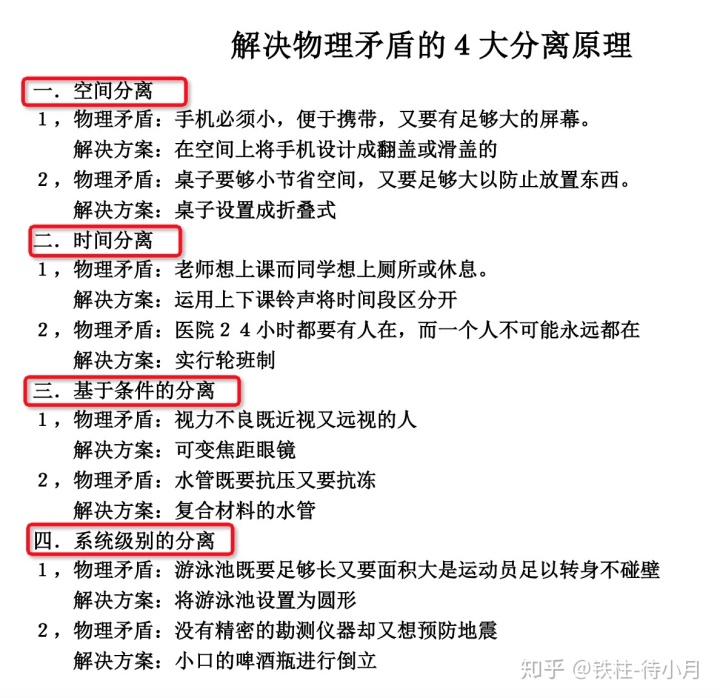展开全文• 前言在解决系统面对高并发的场景下，有三把主要利器，...限流是对稀缺资源访问时，比如秒杀，抢购的商品时，来限制并发和请求的数量，从而有效的进行削峰并使得流量曲线平滑。限流的目的是对并发访问和并发请求进...
• 用JFreeChart绘制光滑曲线，利用最小二乘法数学原理计算，供大家参考，具体内容如下绘制图形：代码：FittingCurve.javapackage org.jevy;import java.util.ArrayList;import java.util.List;import org.jfree.chart....
• 我有一些标记为0或1的数据，我试图使用随机森林预测这些类 ....我正在使用以下代码绘制精确调用和ROC曲线：precision, recall, _ = precision_recall_curve(y_test, y_pred)plt.step(recall, precision, color='b',...
• 作者简介：华哥10年+后端开发工作经验，主要分享：关于java体系的知识，如：java基础知识/数据结算/算法，Spring/MyBatis/Netty源码分析，高并发/高性能/分布式/微服务架构的原理，JVM性能优化等。一致性哈希算法在...
• 利用移动平均滤波器对列向量y进行平滑处理,返回与y等长的列向量yy。移动平均滤波器的默认窗宽为5,yy中元素的计算方法如下:yy(1) = y(1)yy(2)=(y(1) + y(2) + y(3))/3yy(3) = (y(1) 十y(2) 十y(3) + y(4)十y(5))/5yy...
• 如果我想编写一个函数(可能也是一个类),它从不可变的查找表(在调用构造函数时修复)返回线性“平滑”的数据,如下所示：例如func(5.0)== 0.5.>存储查找表的最佳方法是什么？>我在考虑使用两个数组.>还有其他...
• Java学习五分钟系列，目的是为让大家在短时间内搞清楚一项技术的概念、优缺点和适用场景，想要深入的...说到限流，那就要提到限流算法，常用的有【漏桶算法】和【令牌桶算法】两种限流算法。漏桶算法漏桶算法，顾名...
• 先通过一个视频感受一下美格信独创QTrim算法加持下的ANC校准速度眼尖的朋友，是否发现了视频中几大亮点：TWS耳机SPP的连接速度，用时仅3sANC增益的搜索仅用两次扫频(听到3次是因为做了一次被动检测)测试Pa...
• 今天来说说限流的相关内容，包括常见的限流算法、单机限流场景、分布式限流场景以及一些常见限流组件。当然在介绍限流算法和具体场景之前我们先得明确什么是限流，为什么要限流？。任何技术都要搞清它的来源，技术的...
• C#图谱曲线平滑滤波算法，几个平滑直接用。其实平滑算法是触类旁通的。
• 很多文章在谈及曲线平滑的时候，习惯使用拟合的概念，我认为这是不恰当的。平滑后的曲线，一定经过原始的数据点，而拟合曲线，则不一定要经过原始数据点。 一般而言，需要平滑的数据分为两种：时间序列的单值数据、...
• 主要介绍了python基于三阶贝塞尔曲线的数据平滑算法,文中通过示例代码介绍的非常详细，对大家的学习或者工作具有一定的参考学习价值，需要的朋友们下面随着小编来一起学习学习吧
• 一种比较容易做出的是对鼠标移动轨迹画点，再将两点之间以直线相连，最后再进行平滑处理，这种方案不需要什么算法支持，但同样，它面对一个性能和美观的抉择，打的点多，密集，性能相对较低，但更加美观，视觉上更...
• 前言很多文章在谈及曲线平滑的时候，习惯使用拟合的概念，我认为这是不恰当的。平滑后的曲线，一定经过原始的数据点，而拟合曲线，则不一定要经过原始数据点。一般而言，需要平滑的数据分为两种：时间序列的单值数据...
• ## 平滑曲线算法研究

千次阅读 2012-06-02 00:27:58
在绘图术语中样条是通过一组指定点集而生成平滑曲线的柔性带 。 术语 样条曲线 spline curve 绘制样条曲线的方法是给定一组称为控制点的坐标点，可以得到一条样条曲线。 样条曲线分为: 1 插值样条曲线...delete interface null file
• 二次指数平滑法求预测值/*** 二次指数平滑法求预测值* @param list 基础数据集合* @param year 未来第几期* @param modulus 平滑系数* @return 预测值*/private static Double getExpect(List list, int year, ...
• 本附件是一个Excel文档 ...里面的公开的VBA代码可以计算贝塞尔曲线的任意插值，即EXCEL画平滑曲线散点图的方法 附件并详细描述了这一算法，用户可以自行在其他语言实现。 本附件原创作者为 EXCELHOME.NET 的 海底眼
• 用CVI编写个小东西时，发现曲线的拟合已经到了1秒多才能完成一次曲线的拟合。 代码如下： static void curveFitt (double *pdst,const double *psrc,int len) { const double wind_w=0.4; double mypower = pow...
• 2D 折线顶点平滑算法—— 平滑折线通常可以通过两种方式： 通过插值，即在新的平滑曲线上，原始的折线点仍保持不变； 通过近似，这意味着新的平滑曲线将近似原始的折线，但原始点不会被保留下来。 本代码对两种...
• ## 路径平滑算法汇总

千次阅读 2020-02-10 16:44:44
全局路径规划（例如A*算法算法计算路径完毕后，...路径平滑处理的相关算法有：佛洛依德算法 、贝塞尔曲线 佛洛依德算法 https://www.cnblogs.com/miaolegemi/archive/2017/12/05/7986335.html 贝塞尔曲线 https:/......